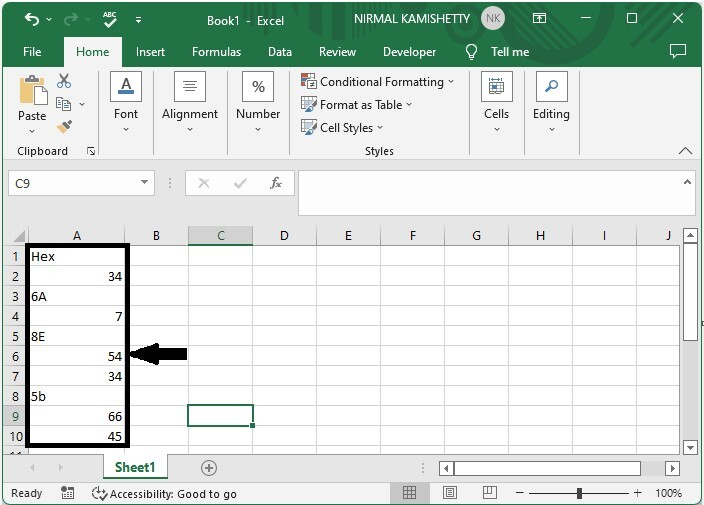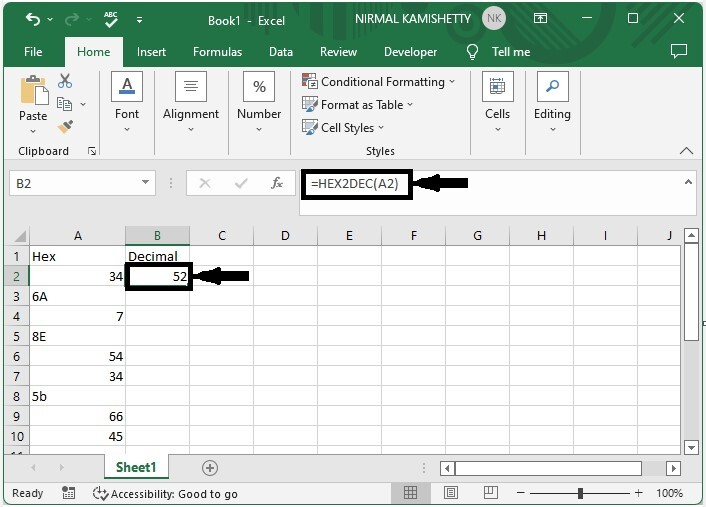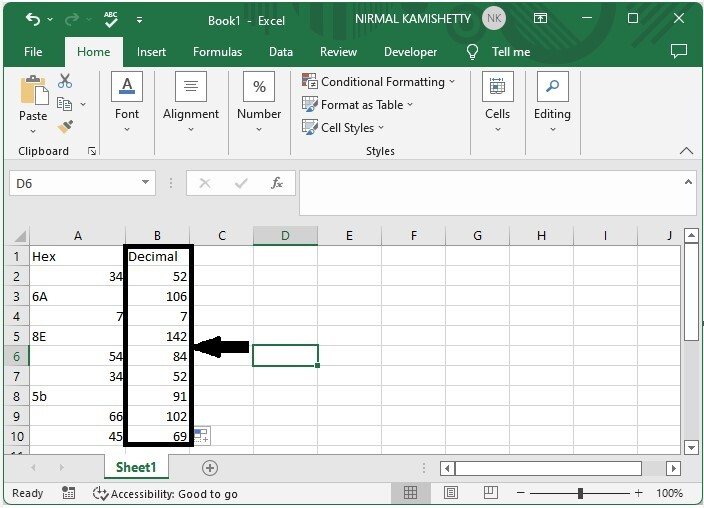# How to Convert Hex Numbers to Decimal Numbers in Excel?

Numbers can be classified into four types: decimal, binary, octal, and hexadecimal. The base values of binary numbers, decimal numbers, octal numbers, and hex numbers are 2, 10, 8, and 16 respectively. We can convert a hex number to a decimal number using formulas in Excel.

A hex number has a base value of 16, and the base values are 0–9 and A–F alphabets. A decimal number has a base of 10, and the base values are 0-9. The base values of an octal number are 0-7. In this tutorial, we will show how you can convert hexadecimal numbers to decimal numbers in Excel and vice versa.

## How To Convert Hex Number to Decimal Number

Here we will use the formula to get the first result, then use the auto-fill handle to get all the results. Let us look at a simple procedure for converting a hexadecimal number to a decimal number in Excel using formulas.

### Step 1:

Consider an Excel sheet with a list of hexadecimal numbers similar to the image below.Now click on the empty cell in our case, cell B2, and enter the formula as =HEX2DEC(A2) and click Enter to get our first result, as shown in the below image.### Step 2

Now to get all the results, drag down from the first result using the auto-fill handle, and our final result will be similar to the below image.We can use the formula =DEC2HEX(A2) to convert any decimal number to a hex number. In the formula, A2 is the address of our values.

Note − In the formula, we can use any combination to convert our values. The first name is our existing number, and the second name is the number we are converting.

• BIN – Binary